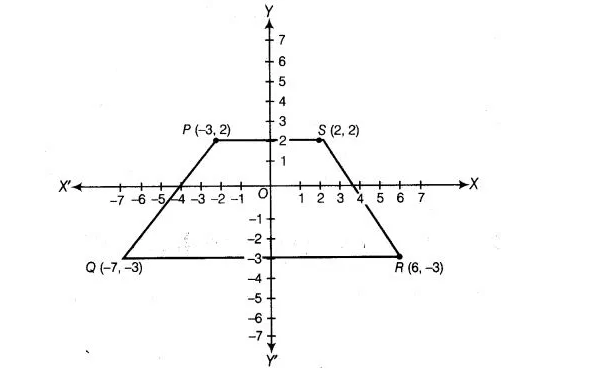# Plot the following points and write the name`
Question:

Plot the following points and write the name of the figure obtained by joining, them in order P(-3, 2), Q(-7, -3), R(6, -3)andS(2, 2).

Solution:

Let X’ OX and Y’ OY be the coordinate axes and mark point on it. Here, point P(-3,2) lies in II quadrant, Q(-7,-3) lies in III quadrant, R(6, – 3) lies in IV quadrant and S(2,2) lies in I quadrant. Plotting the points on the graph paper, the figure obtained is trapezium PQRS.